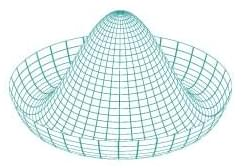There will be a conserved ccurrent for each group generator $a$. Each will result in a conserved charge (that is, an integral of motion). To see this, take in spacetime a volume unbounded in the space-like direction, but limited in time by two space-like surfaces $w_1$ and $w_2$. Integrating $\partial_{\mu} J^{\mu}_a=0$ over this volume, we get an integral over the boundary surface, composed of $w_1$, $w_2$ and the time-like boundaries supposed to be at infinity. If we now suppose the current to be zero at infinity on these boundaries, we remain with.
\int_{w_1}d\sigma_{\mu} J^{\mu}_a=\int_{w_2}d\sigma_{\mu} J^{